# Background¶

The sinking of the RMS Titanic is one of the most infamous shipwrecks in history. On April 15, 1912, during her maiden voyage, the Titanic sank after colliding with an iceberg, killing 1502 out of 2224 passengers and crew. This sensational tragedy shocked the international community and led to better safety regulations for ships.

One of the reasons that the shipwreck led to such loss of life was that there were not enough lifeboats for the passengers and crew. Although there was some element of luck involved in surviving the sinking, some groups of people were more likely to survive than others, such as women, children, and the upper-class.

Complete the analysis of what sorts of people were likely to survive. In particular, we ask you to apply the tools of machine learning to predict which passengers survived the tragedy.

In :
# Suppress Future Warnings
import warnings
warnings.filterwarnings('ignore')


# Data Importing¶¶

In :
import sklearn
import numpy as np
import pandas as pd
import matplotlib
import platform
%config IPCompleter.greedy=True #autocomplete code
message="        Versions        "
print("*"*len(message))
print(message)
print("*"*len(message))
print("Scikit-learn version={}".format(sklearn.__version__))
print("Numpy version={}".format(np.__version__))
print("Pandas version={}".format(pd.__version__))
print("Matplotlib version={}".format(matplotlib.__version__))
print("Python version={}".format(platform.python_version()))

************************
Versions
************************
Scikit-learn version=0.21.3
Numpy version=1.16.5
Pandas version=0.25.1
Matplotlib version=3.1.1
Python version=3.7.4

In :
from sklearn import datasets
from matplotlib import pyplot as plt
import seaborn as sns
%matplotlib inline

In :
# Import our libraries
import pandas as pd
import numpy as np
# Import sklearn libraries
from sklearn.model_selection import train_test_split, GridSearchCV, StratifiedKFold, cross_val_score
from sklearn.model_selection import cross_validate
from sklearn.metrics import accuracy_score, precision_score, recall_score, roc_curve, precision_recall_curve, auc, make_scorer, confusion_matrix, f1_score, fbeta_score
# Import the Naive Bayes, logistic regression, Bagging, RandomForest, AdaBoost, GradientBoost, Decision Trees and SVM Classifier
from sklearn.naive_bayes import MultinomialNB
from sklearn.linear_model import LogisticRegression
from sklearn.tree import DecisionTreeClassifier
from sklearn import svm
from xgboost import XGBClassifier
import seaborn as sns
import matplotlib.pyplot as plt
%matplotlib inline
plt.style.use('seaborn-notebook')
from matplotlib.ticker import StrMethodFormatter
from sklearn.preprocessing import StandardScaler, MinMaxScaler, LabelBinarizer

In :
titanic = pd.read_csv("TitanicDataset_train.csv")
titanic.head(10) #display the first 3 sets of data in train csv

Out:
PassengerId Survived Pclass Name Sex Age SibSp Parch Ticket Fare Cabin Embarked
0 1 0 3 Braund, Mr. Owen Harris male 22.0 1 0 A/5 21171 7.2500 NaN S
1 2 1 1 Cumings, Mrs. John Bradley (Florence Briggs Th... female 38.0 1 0 PC 17599 71.2833 C85 C
2 3 1 3 Heikkinen, Miss. Laina female 26.0 0 0 STON/O2. 3101282 7.9250 NaN S
3 4 1 1 Futrelle, Mrs. Jacques Heath (Lily May Peel) female 35.0 1 0 113803 53.1000 C123 S
4 5 0 3 Allen, Mr. William Henry male 35.0 0 0 373450 8.0500 NaN S
5 6 0 3 Moran, Mr. James male NaN 0 0 330877 8.4583 NaN Q
6 7 0 1 McCarthy, Mr. Timothy J male 54.0 0 0 17463 51.8625 E46 S
7 8 0 3 Palsson, Master. Gosta Leonard male 2.0 3 1 349909 21.0750 NaN S
8 9 1 3 Johnson, Mrs. Oscar W (Elisabeth Vilhelmina Berg) female 27.0 0 2 347742 11.1333 NaN S
9 10 1 2 Nasser, Mrs. Nicholas (Adele Achem) female 14.0 1 0 237736 30.0708 NaN C
In :
titanictest = pd.read_csv("TitanicDataset_test.csv")
titanictest.head(5) #display the first 3 sets of data in train csv

Out:
PassengerId Pclass Name Sex Age SibSp Parch Ticket Fare Cabin Embarked
0 892 3 Kelly, Mr. James male 34.5 0 0 330911 7.8292 NaN Q
1 893 3 Wilkes, Mrs. James (Ellen Needs) female 47.0 1 0 363272 7.0000 NaN S
2 894 2 Myles, Mr. Thomas Francis male 62.0 0 0 240276 9.6875 NaN Q
3 895 3 Wirz, Mr. Albert male 27.0 0 0 315154 8.6625 NaN S
4 896 3 Hirvonen, Mrs. Alexander (Helga E Lindqvist) female 22.0 1 1 3101298 12.2875 NaN S
In :
kaggle_testset = pd.read_csv("100-Acc.csv")

Out:
PassengerId Survived
0 892 0
1 893 1
2 894 0
3 895 0
4 896 1

# Exploratory Data Analysis¶

In :
titanic.info()

<class 'pandas.core.frame.DataFrame'>
RangeIndex: 891 entries, 0 to 890
Data columns (total 12 columns):
PassengerId    891 non-null int64
Survived       891 non-null int64
Pclass         891 non-null int64
Name           891 non-null object
Sex            891 non-null object
Age            714 non-null float64
SibSp          891 non-null int64
Parch          891 non-null int64
Ticket         891 non-null object
Fare           891 non-null float64
Cabin          204 non-null object
Embarked       889 non-null object
dtypes: float64(2), int64(5), object(5)
memory usage: 83.7+ KB

In :
# List out all variables with nulls/missing values
titanic.isnull().sum()

Out:
PassengerId      0
Survived         0
Pclass           0
Name             0
Sex              0
Age            177
SibSp            0
Parch            0
Ticket           0
Fare             0
Cabin          687
Embarked         2
dtype: int64
In :
# Get list of numeric and nonnumeric variables
numvars = list(titanic.columns[titanic.dtypes != "object"])
nonnumvars = list(titanic.columns[titanic.dtypes == "object"])
print(numvars)
print(nonnumvars)

['PassengerId', 'Survived', 'Pclass', 'Age', 'SibSp', 'Parch', 'Fare']
['Name', 'Sex', 'Ticket', 'Cabin', 'Embarked']

In :
# Do some further exploration on list to get list of features used
numvars.remove('PassengerId')
numvars.remove('Survived')
numfeats = numvars
print(numfeats)

#nonnumvars.remove('Cabin')
nonnumvars.remove('Name')
nonnumvars.remove('Ticket')
nonnumfeats = nonnumvars
print(nonnumfeats)

['Pclass', 'Age', 'SibSp', 'Parch', 'Fare']
['Sex', 'Cabin', 'Embarked']

In :
%matplotlib inline
import seaborn as sns
sns.set(style="whitegrid")
ax = sns.boxplot(y=titanic["Age"])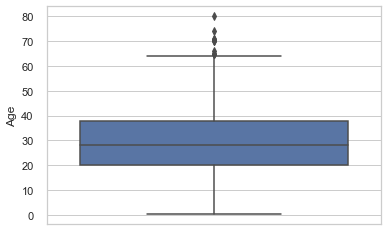In :
titanic['Age'].hist(bins=10)

Out:
<matplotlib.axes._subplots.AxesSubplot at 0x297337bebc8>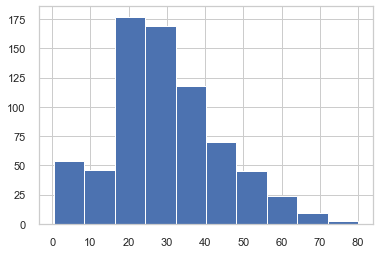# Data Preparation¶

## Handle missing values¶

In :
# Age has some missing values which needs to be filled
titanic['Age'].fillna(titanic['Age'].mean(), inplace=True)
model_mean_age = titanic['Age'].mean()
titanic.describe()

Out:
PassengerId Survived Pclass Age SibSp Parch Fare
count 891.000000 891.000000 891.000000 891.000000 891.000000 891.000000 891.000000
mean 446.000000 0.383838 2.308642 29.699118 0.523008 0.381594 32.204208
std 257.353842 0.486592 0.836071 13.002015 1.102743 0.806057 49.693429
min 1.000000 0.000000 1.000000 0.420000 0.000000 0.000000 0.000000
25% 223.500000 0.000000 2.000000 22.000000 0.000000 0.000000 7.910400
50% 446.000000 0.000000 3.000000 29.699118 0.000000 0.000000 14.454200
75% 668.500000 1.000000 3.000000 35.000000 1.000000 0.000000 31.000000
max 891.000000 1.000000 3.000000 80.000000 8.000000 6.000000 512.329200
In :
# For the cabin parameter there are over 600 missing values
# We will replace the Cabin value with No if missing and Yes if there is a cabin number
titanic['Cabin'].fillna('No', inplace=True)
titanic['Cabin'].replace(regex=r'^((?!No).)*$',value='Yes',inplace=True) titanic.head(2)  Out: PassengerId Survived Pclass Name Sex Age SibSp Parch Ticket Fare Cabin Embarked 0 1 0 3 Braund, Mr. Owen Harris male 22.0 1 0 A/5 21171 7.2500 No S 1 2 1 1 Cumings, Mrs. John Bradley (Florence Briggs Th... female 38.0 1 0 PC 17599 71.2833 Yes C In : # 2 missing values in the embarked # use the mode to replace it titanic['Embarked'].fillna(titanic['Embarked'].mode(), inplace=True) model_embarked_mode = titanic['Embarked'].mode() titanic.head(2)  Out: PassengerId Survived Pclass Name Sex Age SibSp Parch Ticket Fare Cabin Embarked 0 1 0 3 Braund, Mr. Owen Harris male 22.0 1 0 A/5 21171 7.2500 No S 1 2 1 1 Cumings, Mrs. John Bradley (Florence Briggs Th... female 38.0 1 0 PC 17599 71.2833 Yes C In : # Drop the PassengerId titanic = titanic.drop(["PassengerId","Name","Ticket"],axis=1) titanic.head(2)  Out: Survived Pclass Sex Age SibSp Parch Fare Cabin Embarked 0 0 3 male 22.0 1 0 7.2500 No S 1 1 1 female 38.0 1 0 71.2833 Yes C In : # Encode all the categorical variables titanicdf = pd.get_dummies(titanic,columns=nonnumfeats) titanicdf.head(2)  Out: Survived Pclass Age SibSp Parch Fare Sex_female Sex_male Cabin_No Cabin_Yes Embarked_C Embarked_Q Embarked_S 0 0 3 22.0 1 0 7.2500 0 1 1 0 0 0 1 1 1 1 38.0 1 0 71.2833 1 0 0 1 1 0 0 In : titanicdf.dtypes  Out: Survived int64 Pclass int64 Age float64 SibSp int64 Parch int64 Fare float64 Sex_female uint8 Sex_male uint8 Cabin_No uint8 Cabin_Yes uint8 Embarked_C uint8 Embarked_Q uint8 Embarked_S uint8 dtype: object In : titanicdf.describe()  Out: Survived Pclass Age SibSp Parch Fare Sex_female Sex_male Cabin_No Cabin_Yes Embarked_C Embarked_Q Embarked_S count 891.000000 891.000000 891.000000 891.000000 891.000000 891.000000 891.000000 891.000000 891.000000 891.000000 891.000000 891.000000 891.000000 mean 0.383838 2.308642 29.699118 0.523008 0.381594 32.204208 0.352413 0.647587 0.771044 0.228956 0.188552 0.086420 0.725028 std 0.486592 0.836071 13.002015 1.102743 0.806057 49.693429 0.477990 0.477990 0.420397 0.420397 0.391372 0.281141 0.446751 min 0.000000 1.000000 0.420000 0.000000 0.000000 0.000000 0.000000 0.000000 0.000000 0.000000 0.000000 0.000000 0.000000 25% 0.000000 2.000000 22.000000 0.000000 0.000000 7.910400 0.000000 0.000000 1.000000 0.000000 0.000000 0.000000 0.000000 50% 0.000000 3.000000 29.699118 0.000000 0.000000 14.454200 0.000000 1.000000 1.000000 0.000000 0.000000 0.000000 1.000000 75% 1.000000 3.000000 35.000000 1.000000 0.000000 31.000000 1.000000 1.000000 1.000000 0.000000 0.000000 0.000000 1.000000 max 1.000000 3.000000 80.000000 8.000000 6.000000 512.329200 1.000000 1.000000 1.000000 1.000000 1.000000 1.000000 1.000000 In : # Since all values are numeric, do a correction and sort to determine the most important features relative to Survived corr = titanicdf.corr() corr.sort_values(["Survived"], ascending = False, inplace = True) print(corr.Survived)  Survived 1.000000 Sex_female 0.543351 Cabin_Yes 0.316912 Fare 0.257307 Embarked_C 0.168240 Parch 0.081629 Embarked_Q 0.003650 SibSp -0.035322 Age -0.069809 Embarked_S -0.149683 Cabin_No -0.316912 Pclass -0.338481 Sex_male -0.543351 Name: Survived, dtype: float64  # Train Model¶ ## Split Data into Train and Test Sets¶ In : from sklearn.model_selection import train_test_split y = titanicdf["Survived"].values X = titanicdf.drop(["Survived"],axis=1).values X_train, X_test, y_train, y_test = train_test_split(X, y, test_size=0.1)  ## Train Model with Algorithm: Logistics Regression¶ In : # Train Model with Logistics Regression from sklearn.linear_model import LogisticRegression LogisticRegression_Model = LogisticRegression() LogisticRegression_Model.fit(X_train,y_train) Y_prediction = LogisticRegression_Model.predict(X_test) LogisticRegression_Model.score(X_train, y_train) acc_LR = round(LogisticRegression_Model.score(X_train, y_train) * 100, 2)  ## Train Model with Algorithm: CART Classification Tree¶ In : from sklearn.tree import DecisionTreeClassifier CART_Model = DecisionTreeClassifier() CART_Model.fit(X_train,y_train) Y_prediction = CART_Model.predict(X_test) CART_Model.score(X_train, y_train) acc_CART = round(CART_Model.score(X_train, y_train) * 100, 2)  ## Train Model with Algorithm: SVM¶ In : from sklearn.svm import SVC SVM_Model = SVC() SVM_Model.fit(X_train, y_train) Y_prediction = SVM_Model.predict(X_test) SVM_Model.score(X_train, y_train) acc_SVM = round(SVM_Model.score(X_train, y_train) * 100, 2)  ## Train Model with Algorithm: K-Nearest Neighbour¶ In : from sklearn.neighbors import KNeighborsClassifier K_NearestNeighbour = np.arange(1,20) train_accuracy = np.empty(len(K_NearestNeighbour)) test_accuracy = np.empty(len(K_NearestNeighbour)) #Loop over the different values of K for i,k in enumerate(K_NearestNeighbour): #Setup K-NN classifier knn_classifier = KNeighborsClassifier(n_neighbors = k) #train the model knn_classifier.fit(X_train, y_train) #Compute accuracy of training set train_accuracy[i] = knn_classifier.score(X_train, y_train) #Compute accuracy of test set test_accuracy[i] = knn_classifier.score(X_test, y_test)  Checking accuracy of K In : plt.title('K-NN varying number of neighbors') plt.plot(K_NearestNeighbour, test_accuracy, label = 'Testing Accuracy') plt.plot(K_NearestNeighbour, train_accuracy, label = 'Training Accuracy') plt.legend() plt.xlabel('No. of neighbors') plt.ylabel('Accuracy') plt.show()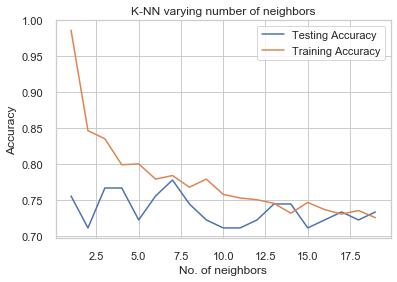From the above graph, I will re-run the test, however I will limit it down to below 9 In : K_NearestNeighbour_run2 = np.arange(1,9) train_accuracy = np.empty(len(K_NearestNeighbour_run2)) test_accuracy = np.empty(len(K_NearestNeighbour_run2)) #Loop over the different values of K for i,k in enumerate(K_NearestNeighbour_run2): #Setup K-NN classifier knn_classifier = KNeighborsClassifier(n_neighbors = k) #train the model knn_classifier.fit(X_train, y_train) #Compute accuracy of training set train_accuracy[i] = knn_classifier.score(X_train, y_train) #Compute accuracy of test set test_accuracy[i] = knn_classifier.score(X_test, y_test)  In : plt.title('K-NN varying number of neighbors') plt.plot(K_NearestNeighbour_run2 , test_accuracy, label = 'Testing Accuracy') plt.plot(K_NearestNeighbour_run2 , train_accuracy, label = 'Training Accuracy') plt.legend() plt.xlabel('No. of neighbors') plt.ylabel('Accuracy') plt.show()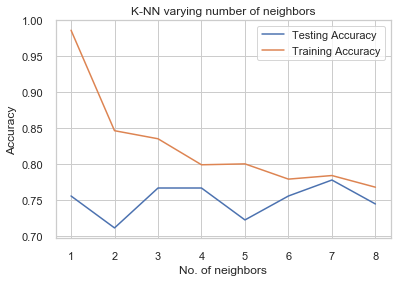It seems like we have a good accuracy with 2 so I will take that as my K value In : K_NearestNeighbour_Model = KNeighborsClassifier(n_neighbors = 2) K_NearestNeighbour_Model.fit(X_train, y_train) Y_prediction = K_NearestNeighbour_Model.predict(X_test) K_NearestNeighbour_Model.score(X_train, y_train) acc_KNN = round(K_NearestNeighbour_Model.score(X_train, y_train) * 100, 2)  ## Train Model with Algorithm: Naive Bayes¶ In : from sklearn import metrics from sklearn.naive_bayes import GaussianNB from sklearn.metrics import confusion_matrix from sklearn.metrics import classification_report from sklearn.metrics import roc_curve from sklearn.metrics import roc_auc_score NaiveBayes_Model = GaussianNB() NaiveBayes_Model.fit(X_train, y_train) Y_prediction = NaiveBayes_Model.predict(X_test) NaiveBayes_Model.score(X_train, y_train) acc_NB = round(NaiveBayes_Model.score(X_train, y_train) * 100, 2)  ## Train Model with Algorithm: Stochastic Gradient Descent¶ In : from sklearn.linear_model import SGDClassifier SGD_Model = SGDClassifier() SGD_Model.fit(X_train, y_train) Y_prediction = SGD_Model.predict(X_test) SGD_Model.score(X_train, y_train) acc_SGD = round(SGD_Model.score(X_train, y_train) * 100, 2)  ## Train Model with Algorithm: Gradient Boosting Classifier¶ In : from sklearn.ensemble import GradientBoostingClassifier from sklearn.metrics import classification_report, confusion_matrix, roc_curve, auc learning_rates = [0.1, 0.2, 0.3, 0.4, 0.45, 0.5, 0.6, 0.75, 0.8, 1] for learning_rate in learning_rates: gb = GradientBoostingClassifier(n_estimators=63, learning_rate = learning_rate, max_features=2, max_depth = 5, random_state = 0) gb.fit(X_train, y_train) print("Learning rate: ", learning_rate) print("Accuracy score (training): {0:.3f}".format(gb.score(X_train, y_train))) print("Accuracy score (validation): {0:.3f}".format(gb.score(X_test, y_test))) print()  Learning rate: 0.1 Accuracy score (training): 0.905 Accuracy score (validation): 0.811 Learning rate: 0.2 Accuracy score (training): 0.933 Accuracy score (validation): 0.811 Learning rate: 0.3 Accuracy score (training): 0.950 Accuracy score (validation): 0.811 Learning rate: 0.4 Accuracy score (training): 0.964 Accuracy score (validation): 0.811 Learning rate: 0.45 Accuracy score (training): 0.970 Accuracy score (validation): 0.756 Learning rate: 0.5 Accuracy score (training): 0.969 Accuracy score (validation): 0.778 Learning rate: 0.6 Accuracy score (training): 0.975 Accuracy score (validation): 0.756 Learning rate: 0.75 Accuracy score (training): 0.981 Accuracy score (validation): 0.789 Learning rate: 0.8 Accuracy score (training): 0.978 Accuracy score (validation): 0.811 Learning rate: 1 Accuracy score (training): 0.954 Accuracy score (validation): 0.822  As you can see, the learning rate at 0.5 gave the best score so I will use that to train In : GB_Model = GradientBoostingClassifier(n_estimators=63, learning_rate = 0.5, max_features=2, max_depth = 5, random_state = 0) GB_Model.fit(X_train, y_train) Y_prediction = GB_Model.predict(X_test) GB_Model.score(X_train, y_train) acc_GBC = round(GB_Model.score(X_train, y_train) * 100, 2)  ## Train Model with Algorithm: Random Forest¶ In : random_forest = RandomForestClassifier(n_estimators=100) random_forest.fit(X_train, y_train) Y_prediction = random_forest.predict(X_test) random_forest.score(X_train, y_train) acc_random_forest = round(random_forest.score(X_train, y_train) * 100, 2)  ## Train model with Algorithm: Perceptron¶ In : from sklearn.linear_model import Perceptron perceptron = Perceptron(max_iter=5) perceptron.fit(X_train, y_train) Y_pred = perceptron.predict(X_test) acc_perceptron = round(perceptron.score(X_train, y_train) * 100, 2)  ## Train model with Algorithm: Linear Support Vector Machine¶ In : from sklearn.svm import LinearSVC linear_svc = LinearSVC() linear_svc.fit(X_train, y_train) Y_pred = linear_svc.predict(X_test) acc_linear_svc = round(linear_svc.score(X_train, y_train) * 100, 2)  # Score and Evaluate Model¶ ## Score Model and Evaluate Model with: Logistics Regression¶ In : results = pd.DataFrame({ 'Model': ['Linear Regression', 'CART','SVM', 'KNN', 'Naive Bayes','SGD','GBC', 'Random Forest','Perceptron','LSVM'], 'Score': [acc_LR,acc_CART,acc_SVM,acc_KNN,acc_NB,acc_SGD, acc_GBC, acc_random_forest, acc_perceptron, acc_linear_svc]}) result_df = results.sort_values(by='Score', ascending=False) result_df = result_df.set_index('Score') result_df.head(10)  Out: Model Score 98.63 CART 98.63 Random Forest 96.88 GBC 88.51 SVM 84.64 KNN 79.15 Linear Regression 77.28 Naive Bayes 76.78 LSVM 75.66 SGD 71.16 Perceptron In : # score model for test set y_hat_LogisticRegression_Model = LogisticRegression_Model.predict(X_test)  In : from sklearn.metrics import confusion_matrix # evaluate model for test set class_names=["Survived","Died"] cm = confusion_matrix(y_test, y_hat_LogisticRegression_Model, labels=[1,0]) df_cm = pd.DataFrame(cm, columns=class_names, index = class_names) df_cm.index.name = 'Actual' df_cm.columns.name = 'Predicted' plt.figure(figsize = (6,5)) sns.set(font_scale=1.4)#for label size sns.heatmap(df_cm, cmap="Blues", annot=True,annot_kws={"size": 16})# font size  Out: <matplotlib.axes._subplots.AxesSubplot at 0x29733a17188>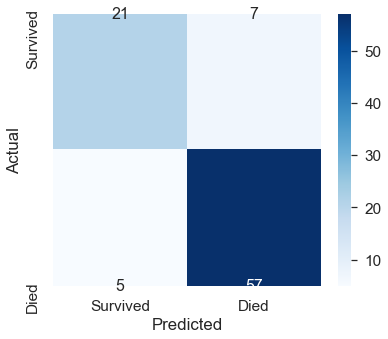In : # Accuracy score for test set from sklearn.metrics import accuracy_score score = accuracy_score(y_test, y_hat_LogisticRegression_Model) print("Accuracy score for the test set with Logistics Regression={:.2f}%".format(score*100))  Accuracy score for the test set with Logistics Regression=86.67%  ## Score Model and Evaluate Model with: CART¶ In : # score model for test set y_hat_CART_Model = CART_Model.predict(X_test)  In : # evaluate model for test set class_names=["Survived","Died"] cm = confusion_matrix(y_test, y_hat_CART_Model, labels=[1,0]) df_cm = pd.DataFrame(cm, columns=class_names, index = class_names) df_cm.index.name = 'Actual' df_cm.columns.name = 'Predicted' plt.figure(figsize = (6,5)) sns.set(font_scale=1)#for label size sns.heatmap(df_cm, cmap="Blues", annot=True,annot_kws={"size": 16})# font size  Out: <matplotlib.axes._subplots.AxesSubplot at 0x29733ad71c8>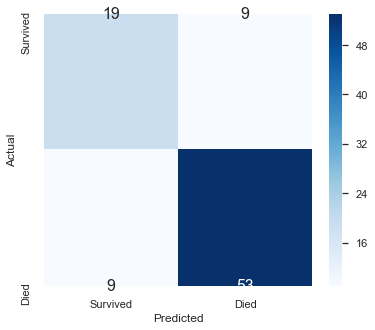In : # Accuracy score for test set from sklearn.metrics import accuracy_score score = accuracy_score(y_test, y_hat_CART_Model) print("Accuracy score for the test set with CART={:.2f}%".format(score*100))  Accuracy score for the test set with CART=80.00%  ## Score Model and Evaluate Model with: SVM¶ In : # score model for test set y_hat_SVM_Model = SVM_Model.predict(X_test)  In : # evaluate model for test set class_names=["Survived","Died"] cm = confusion_matrix(y_test, y_hat_SVM_Model, labels=[1,0]) df_cm = pd.DataFrame(cm, columns=class_names, index = class_names) df_cm.index.name = 'Actual' df_cm.columns.name = 'Predicted' plt.figure(figsize = (6,5)) sns.set(font_scale=1)#for label size sns.heatmap(df_cm, cmap="Blues", annot=True,annot_kws={"size": 16})# font size  Out: <matplotlib.axes._subplots.AxesSubplot at 0x297339e5288>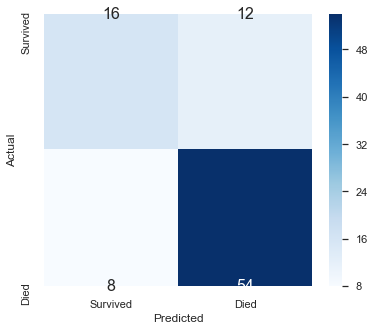In : # Accuracy score for test set from sklearn.metrics import accuracy_score score = accuracy_score(y_test, y_hat_SVM_Model) print("Accuracy score for the test set with SVM={:.2f}%".format(score*100))  Accuracy score for the test set with SVM=77.78%  ## Score Model and Evaluate Model with: K-Nearest Neighbour¶ In : y_hat_KNN_Model = K_NearestNeighbour_Model.predict(X_test)  In : # evaluate model for test set class_names=["Survived","Died"] cm = confusion_matrix(y_test, y_hat_KNN_Model, labels=[1,0]) df_cm = pd.DataFrame(cm, columns=class_names, index = class_names) df_cm.index.name = 'Actual' df_cm.columns.name = 'Predicted' plt.figure(figsize = (6,5)) sns.set(font_scale=1)#for label size sns.heatmap(df_cm, cmap="Blues", annot=True,annot_kws={"size": 16})# font size  Out: <matplotlib.axes._subplots.AxesSubplot at 0x29734be1fc8>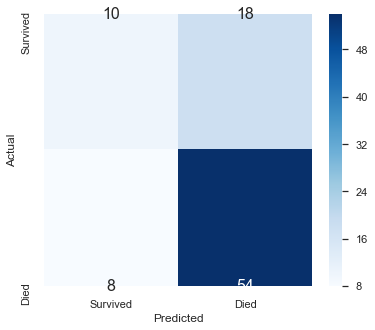In : # Accuracy score for test set from sklearn.metrics import accuracy_score score = accuracy_score(y_test, y_hat_KNN_Model) print("Accuracy score for the test set with KNN={:.2f}%".format(score*100))  Accuracy score for the test set with KNN=71.11%  ## Score Model and Evaluate Model with: Naive Bayes¶ In : y_hat_NB_Model = NaiveBayes_Model.predict(X_test)  In : # evaluate model for test set class_names=["Survived","Died"] cm = confusion_matrix(y_test, y_hat_NB_Model, labels=[1,0]) df_cm = pd.DataFrame(cm, columns=class_names, index = class_names) df_cm.index.name = 'Actual' df_cm.columns.name = 'Predicted' plt.figure(figsize = (6,5)) sns.set(font_scale=1)#for label size sns.heatmap(df_cm, cmap="Blues", annot=True,annot_kws={"size": 16})# font size  Out: <matplotlib.axes._subplots.AxesSubplot at 0x29734ca16c8>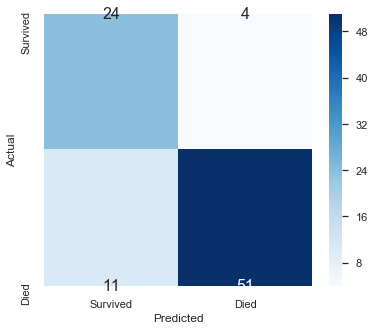In : # Accuracy score for test set from sklearn.metrics import accuracy_score score = accuracy_score(y_test, y_hat_NB_Model) print("Accuracy score for the test set with NB={:.2f}%".format(score*100))  Accuracy score for the test set with NB=83.33%  ## Score Model and Evaluate Model with: Stochastic Gradient Descent¶ In : y_hat_SGD_Model = SGD_Model.predict(X_test)  In : # evaluate model for test set class_names=["Survived","Died"] cm = confusion_matrix(y_test, y_hat_SGD_Model, labels=[1,0]) df_cm = pd.DataFrame(cm, columns=class_names, index = class_names) df_cm.index.name = 'Actual' df_cm.columns.name = 'Predicted' plt.figure(figsize = (6,5)) sns.set(font_scale=1)#for label size sns.heatmap(df_cm, cmap="Blues", annot=True,annot_kws={"size": 16})# font size  Out: <matplotlib.axes._subplots.AxesSubplot at 0x29734ca3c88>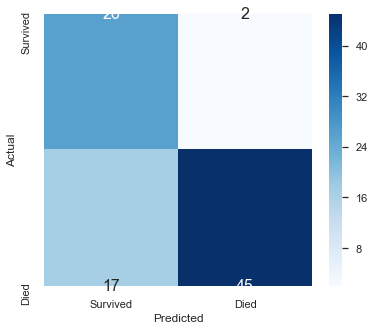In : # Accuracy score for test set from sklearn.metrics import accuracy_score score = accuracy_score(y_test, y_hat_SGD_Model) print("Accuracy score for the test set with SGD={:.2f}%".format(score*100))  Accuracy score for the test set with SGD=78.89%  ## Score Model and Evaluate Model with: Gradient Boosting Classifier¶ In : y_hat_GB_Model = GB_Model.predict(X_test)  In : # evaluate model for test set class_names=["Survived","Died"] cm = confusion_matrix(y_test, y_hat_GB_Model, labels=[1,0]) df_cm = pd.DataFrame(cm, columns=class_names, index = class_names) df_cm.index.name = 'Actual' df_cm.columns.name = 'Predicted' plt.figure(figsize = (6,5)) sns.set(font_scale=1)#for label size sns.heatmap(df_cm, cmap="Blues", annot=True,annot_kws={"size": 16})# font size  Out: <matplotlib.axes._subplots.AxesSubplot at 0x29734de8408>In : # Accuracy score for test set from sklearn.metrics import accuracy_score score = accuracy_score(y_test, y_hat_GB_Model) print("Accuracy score for the test set with GB={:.2f}%".format(score*100))  Accuracy score for the test set with GB=77.78%  ## Score Model and Evaluate Model with: Random Forest¶ In : y_hat_RF_Model = random_forest.predict(X_test)  In : # evaluate model for test set class_names=["Survived","Died"] cm = confusion_matrix(y_test, y_hat_RF_Model, labels=[1,0]) df_cm = pd.DataFrame(cm, columns=class_names, index = class_names) df_cm.index.name = 'Actual' df_cm.columns.name = 'Predicted' plt.figure(figsize = (6,5)) sns.set(font_scale=1)#for label size sns.heatmap(df_cm, cmap="Blues", annot=True,annot_kws={"size": 16})# font size  Out: <matplotlib.axes._subplots.AxesSubplot at 0x29734d5cc88>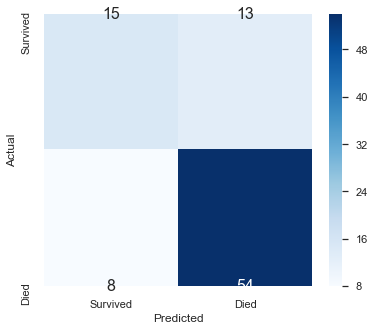In : # Accuracy score for test set from sklearn.metrics import accuracy_score score = accuracy_score(y_test, y_hat_RF_Model) print("Accuracy score for the test set with RF={:.2f}%".format(score*100))  Accuracy score for the test set with RF=76.67%  ## Score Model and Evaluate Model with: Perceptron¶ In : y_hat_Per_Model = perceptron.predict(X_test)  In : # evaluate model for test set class_names=["Survived","Died"] cm = confusion_matrix(y_test, y_hat_Per_Model, labels=[1,0]) df_cm = pd.DataFrame(cm, columns=class_names, index = class_names) df_cm.index.name = 'Actual' df_cm.columns.name = 'Predicted' plt.figure(figsize = (6,5)) sns.set(font_scale=1)#for label size sns.heatmap(df_cm, cmap="Blues", annot=True,annot_kws={"size": 16})# font size  Out: <matplotlib.axes._subplots.AxesSubplot at 0x29734f17148>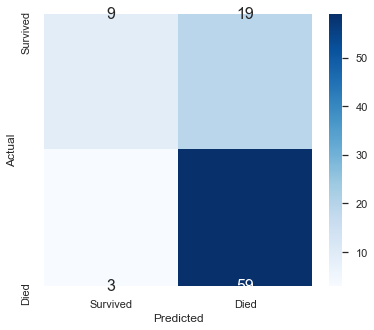In : # Accuracy score for test set from sklearn.metrics import accuracy_score score = accuracy_score(y_test, y_hat_Per_Model) print("Accuracy score for the test set with Per={:.2f}%".format(score*100))  Accuracy score for the test set with Per=75.56%  ## Score Model and Evaluate Model with:LinearSVM¶ In : y_hat_LSVM_Model = linear_svc.predict(X_test)  In : # evaluate model for test set class_names=["Survived","Died"] cm = confusion_matrix(y_test, y_hat_LSVM_Model, labels=[1,0]) df_cm = pd.DataFrame(cm, columns=class_names, index = class_names) df_cm.index.name = 'Actual' df_cm.columns.name = 'Predicted' plt.figure(figsize = (6,5)) sns.set(font_scale=1)#for label size sns.heatmap(df_cm, cmap="Blues", annot=True,annot_kws={"size": 16})# font size  Out: <matplotlib.axes._subplots.AxesSubplot at 0x29733863cc8>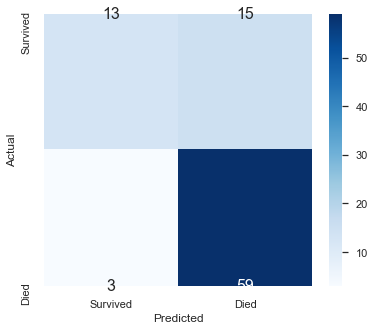In : # Accuracy score for test set from sklearn.metrics import accuracy_score score = accuracy_score(y_test, y_hat_LSVM_Model) print("Accuracy score for the test set with LSVM={:.2f}%".format(score*100))  Accuracy score for the test set with LSVM=80.00%  # Submitting to Kaggle¶ ## Stupid Baseline (Everyone Dies)¶ The stupid baseline is based on the majority of Survived status. In which case, we will have a rule which states that everybody died in the Titanic. In : dfout = pd.DataFrame() dfout[["PassengerId"]] = titanictest[["PassengerId"]] dfout["Survived"] = 0 dfout[:5]  Out: PassengerId Survived 0 892 0 1 893 0 2 894 0 3 895 0 4 896 0 In : dfout.to_csv("stupidbaseline.csv",index=False)  ## Data Preparation for the test.csv¶ In : titanictest.dtypes  Out: PassengerId int64 Pclass int64 Name object Sex object Age float64 SibSp int64 Parch int64 Ticket object Fare float64 Cabin object Embarked object dtype: object In : kaggle_testset.dtypes  Out: PassengerId int64 Survived int64 dtype: object In : titanictestdf = titanictest.drop(["PassengerId","Name","Ticket"],axis=1) titanictestdf.head(2)  Out: Pclass Sex Age SibSp Parch Fare Cabin Embarked 0 3 male 34.5 0 0 7.8292 NaN Q 1 3 female 47.0 1 0 7.0000 NaN S In : kaggle_testset.head(2)  Out: PassengerId Survived 0 892 0 1 893 1 In : # List out all variables with nulls/missing values titanictestdf.isnull().sum()  Out: Pclass 0 Sex 0 Age 86 SibSp 0 Parch 0 Fare 1 Cabin 327 Embarked 0 dtype: int64 In : # fill in the missing age titanictestdf['Age'].fillna(titanic['Age'].mean(), inplace=True)  In : # fill in the missing cabins # We will replace the Cabin value with No if missing and Yes if there is a cabin number titanictestdf['Cabin'].fillna('No', inplace=True) titanictestdf['Cabin'].replace(regex=r'^((?!No).)*$',value='Yes',inplace=True)

Out:
Pclass Sex Age SibSp Parch Fare Cabin Embarked
0 3 male 34.5 0 0 7.8292 No Q
1 3 female 47.0 1 0 7.0000 No S
In :
# fill in the missing fare with the mean fare
titanictestdf['Fare'].fillna(titanic['Fare'].mean(), inplace=True)

In :
# List out all variables with nulls/missing values
titanictestdf.isnull().sum()

Out:
Pclass      0
Sex         0
Age         0
SibSp       0
Parch       0
Fare        0
Cabin       0
Embarked    0
dtype: int64
In :
# Encode all the categorical variables
predictdf = pd.get_dummies(titanictestdf,columns=nonnumfeats)

Out:
Pclass Age SibSp Parch Fare Sex_female Sex_male Cabin_No Cabin_Yes Embarked_C Embarked_Q Embarked_S
0 3 34.5 0 0 7.8292 0 1 1 0 0 1 0
1 3 47.0 1 0 7.0000 1 0 1 0 0 0 1
In :
Xp = predictdf.values
Xp[:2]

Out:
array([[ 3.    , 34.5   ,  0.    ,  0.    ,  7.8292,  0.    ,  1.    ,
1.    ,  0.    ,  0.    ,  1.    ,  0.    ],
[ 3.    , 47.    ,  1.    ,  0.    ,  7.    ,  1.    ,  0.    ,
1.    ,  0.    ,  0.    ,  0.    ,  1.    ]])

## Prediction with Logistics Regression Trained Model¶

In :
yp_hat_LGR = LogisticRegression_Model.predict(Xp)

In :
dfout = pd.DataFrame()
dfout[["PassengerId"]] = titanictest[["PassengerId"]]
dfout["Survived"] = yp_hat_LGR
dfout[:418]

Out:
PassengerId Survived
0 892 0
1 893 0
2 894 0
3 895 0
4 896 1
... ... ...
413 1305 0
414 1306 1
415 1307 0
416 1308 0
417 1309 0

418 rows × 2 columns

In :
dfout.to_csv("Prediction_LogisticRegression.csv",index=False)


## Prediction with CART Trained Model¶

In :
yp_hat_CART = CART_Model.predict(Xp)

In :
dfout = pd.DataFrame()
dfout[["PassengerId"]] = titanictest[["PassengerId"]]
dfout["Survived"] = yp_hat_CART
dfout[:418]

Out:
PassengerId Survived
0 892 0
1 893 0
2 894 1
3 895 1
4 896 1
... ... ...
413 1305 0
414 1306 1
415 1307 0
416 1308 0
417 1309 1

418 rows × 2 columns

In :
dfout.to_csv("Prediction_CART.csv",index=False)


## Prediction with SVM Trained Model¶

In :
yp_hat_SVM = SVM_Model.predict(Xp)

In :
dfout = pd.DataFrame()
dfout[["PassengerId"]] = titanictest[["PassengerId"]]
dfout["Survived"] = yp_hat_SVM
dfout[:418]

Out:
PassengerId Survived
0 892 0
1 893 0
2 894 0
3 895 0
4 896 0
... ... ...
413 1305 0
414 1306 1
415 1307 0
416 1308 0
417 1309 0

418 rows × 2 columns

In :
dfout.to_csv("Prediction_SVM.csv",index=False)


## Prediction with K-Nearest Neighbour Regression Trained Model¶

In :
yp_hat_KNN = K_NearestNeighbour_Model.predict(Xp)

In :
dfout = pd.DataFrame()
dfout[["PassengerId"]] = titanictest[["PassengerId"]]
dfout["Survived"] = yp_hat_KNN
dfout[:418]

Out:
PassengerId Survived
0 892 0
1 893 0
2 894 0
3 895 0
4 896 0
... ... ...
413 1305 0
414 1306 1
415 1307 0
416 1308 0
417 1309 0

418 rows × 2 columns

In :
dfout.to_csv("Prediction_KNN.csv",index=False)


## Prediction with Naive Bayes Trained Model¶

In :
yp_hat_NB = NaiveBayes_Model.predict(Xp)

In :
dfout = pd.DataFrame()
dfout[["PassengerId"]] = titanictest[["PassengerId"]]
dfout["Survived"] = yp_hat_NB
dfout[:418]

Out:
PassengerId Survived
0 892 0
1 893 1
2 894 0
3 895 0
4 896 1
... ... ...
413 1305 0
414 1306 1
415 1307 0
416 1308 0
417 1309 0

418 rows × 2 columns

In :
dfout.to_csv("Prediction_NB.csv",index=False)


## Prediction with Stochastic Gradient Descent Trained Model¶

In :
yp_hat_SGD = SGD_Model.predict(Xp)

In :
dfout = pd.DataFrame()
dfout[["PassengerId"]] = titanictest[["PassengerId"]]
dfout["Survived"] = yp_hat_SGD
dfout[:418]

Out:
PassengerId Survived
0 892 0
1 893 0
2 894 0
3 895 0
4 896 1
... ... ...
413 1305 0
414 1306 1
415 1307 0
416 1308 0
417 1309 0

418 rows × 2 columns

In :
dfout.to_csv("Prediction_SGD.csv",index=False)


## Predicting with Gradient Boosting Classifier¶

In :
yp_hat_GBC = GB_Model.predict(Xp)

In :
dfout = pd.DataFrame()
dfout[["PassengerId"]] = titanictest[["PassengerId"]]
dfout["Survived"] = yp_hat_GBC
dfout[:418]

Out:
PassengerId Survived
0 892 0
1 893 0
2 894 0
3 895 0
4 896 0
... ... ...
413 1305 0
414 1306 1
415 1307 0
416 1308 0
417 1309 1

418 rows × 2 columns

In :
dfout.to_csv("Prediction_GBC.csv",index=False)


## Predicting with Random Forest¶

In :
yp_hat_RF = random_forest.predict(Xp)

In :
dfout = pd.DataFrame()
dfout[["PassengerId"]] = titanictest[["PassengerId"]]
dfout["Survived"] = yp_hat_RF
dfout[:418]

Out:
PassengerId Survived
0 892 0
1 893 0
2 894 0
3 895 1
4 896 0
... ... ...
413 1305 0
414 1306 1
415 1307 0
416 1308 0
417 1309 1

418 rows × 2 columns

In :
dfout.to_csv("Prediction_RF.csv",index=False)


## Predicting with Perceptron¶

In :
yp_hat_Per = perceptron.predict(Xp)

In :
dfout = pd.DataFrame()
dfout[["PassengerId"]] = titanictest[["PassengerId"]]
dfout["Survived"] = yp_hat_Per
dfout[:418]

Out:
PassengerId Survived
0 892 0
1 893 0
2 894 0
3 895 0
4 896 0
... ... ...
413 1305 0
414 1306 1
415 1307 0
416 1308 0
417 1309 0

418 rows × 2 columns

In :
dfout.to_csv("Prediction_Perceptron.csv",index=False)


## Predicting with Linear SVM¶

In :
yp_hat_LSVM = linear_svc.predict(Xp)

In :
dfout = pd.DataFrame()
dfout[["PassengerId"]] = titanictest[["PassengerId"]]
dfout["Survived"] = yp_hat_LSVM
dfout[:418]

Out:
PassengerId Survived
0 892 0
1 893 0
2 894 0
3 895 0
4 896 0
... ... ...
413 1305 0
414 1306 1
415 1307 0
416 1308 0
417 1309 0

418 rows × 2 columns

In :
dfout.to_csv("Prediction_LSVM.csv",index=False)


# Conclusions¶

This project is to predict the who would survive in the titanic, as the ouput is either survived or died, it is a classification task. A regression task would be if the output is scalable, like prices or percentage, where data is on a scale rather than like a yes or no. So unless this task is changed in such a way to predict the chances of survivalbility then, it would be a regression task. Otherwise, it is a classification task.

After running different models, the better performing ones are Linear Regression and Gradient Boosting Classifier On Kaggle, my highest score was a 0.77 which means my prediction are 77% correct.

For the data, I removed the names, id and ticket when training as they should not be the factors of survivability. I did not do any special modifications to the features as I did not see a point in doing so.

For learning algorithms, I felt that the best way to go about it was to use as many as possible and then sifting out the better ones to use. So for example, in my tests, Gradient Boosting Classifier and Logistic Regression were among the top when it come's to accuracy of prediction and hence, these were my choices if I needed to make a prediction. However, I trained so many models is to ensure that I can see which are more accurate.

As for hyperparameters, I did not go into very specific tuning, so for example, I only tuned the K value of the K-NN model, as well as changing the learning rate for the Gradient Boosting Classifier.

I check my results against kaggle for accuracy as the accuracy check I've implemented in Jupyter notebook is not as accurate as the one on Kaggle. When compared to a baseline assumption that everyone died, it was about 15% more accurate. The baseline got a score of 0.62 whereas mine was 0.77.